## Ramashankar Rajppot

teacher | Physics Maths Chemistry Science Computer

### Description

I am a MCA professional with a teaching experience of more than 7 years. I am very passionate about teaching Maths, Physics, Chemistry, Science & Computers all through my career.I extensively uses the quality teaching material with better examples.

MCA

7+ Years

11-12

### Current Organization

DPS School

Atom and Nucleus - Formulas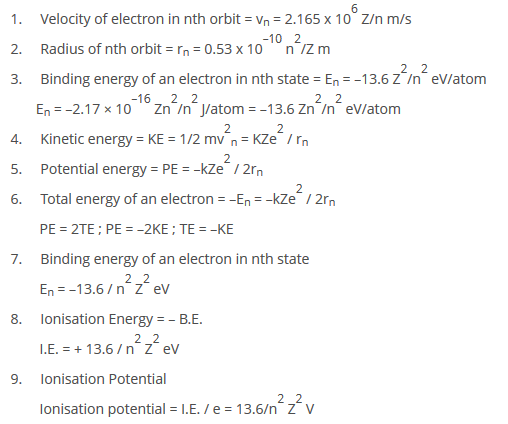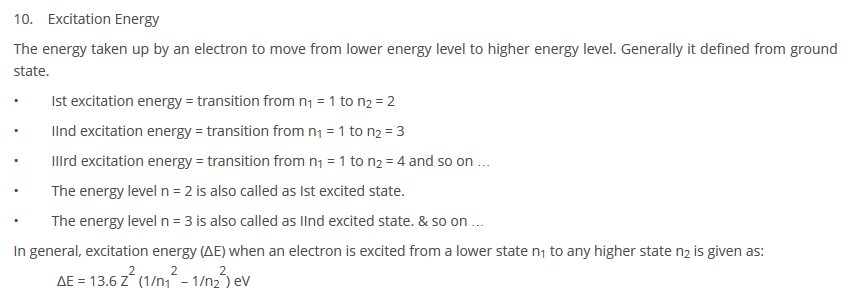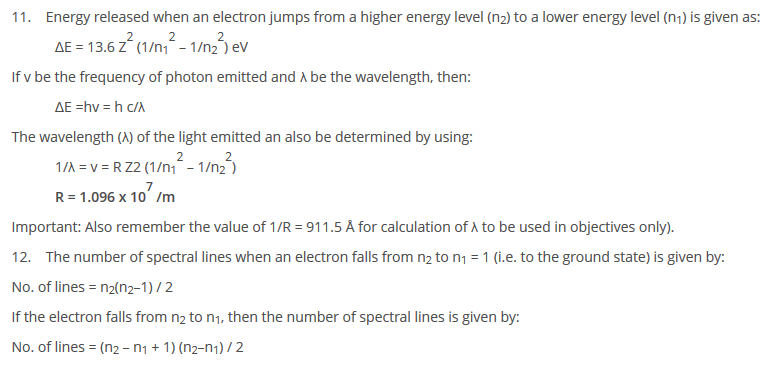Theory of Graph

A graph says how the dependent quantity changes its value with respect to change in vol of independent quantity (variable/parameter).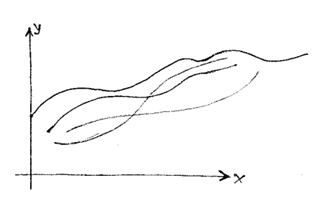Information that we obtain from the graph.

1. Pattern of dependency of one variable on other variable.
2. The nature of dependency, i.e. how a variable in changing with other variable.
3. The rate of change of one variable to other variable at any point.
4. The extreme values of dependent variable.
5. From a graph of a function, we can obtain domain, range, nature, period, etc. of the function.

Domain is the value on X-axis associated with graph.

Range is the value on Y-axis associated with graph.

Use of application of derivatives in Graph Theory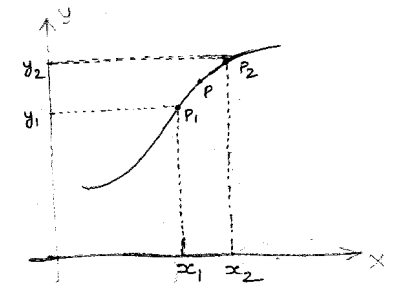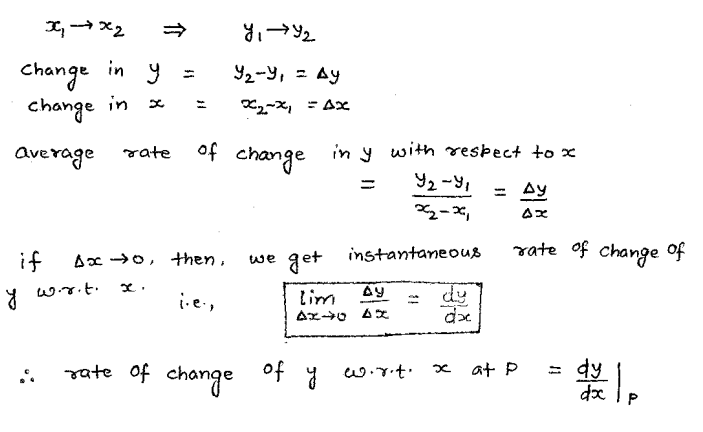Use of application of derivatives in Graph Theory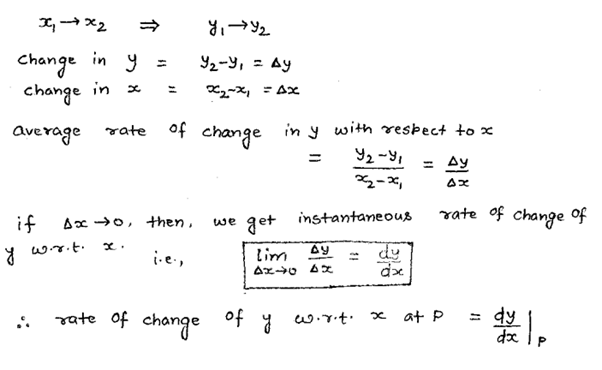Application of “rate of change” in Physics: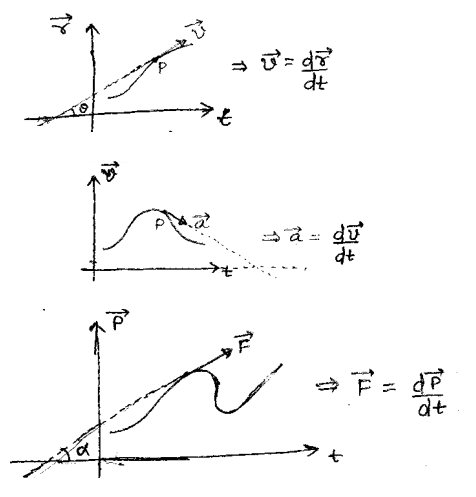To find the “slope of tangent” to the curve: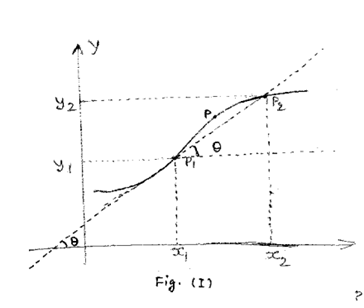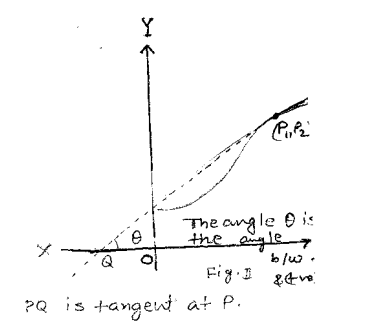Line joining two points on the curve is called chord.

If P1 & P2 become closer and closer together, then at a point P1, P1 & P2 will become adjacent point and then the chord will be known as tangent.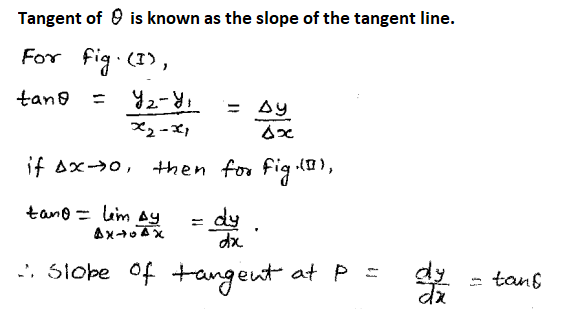Solid States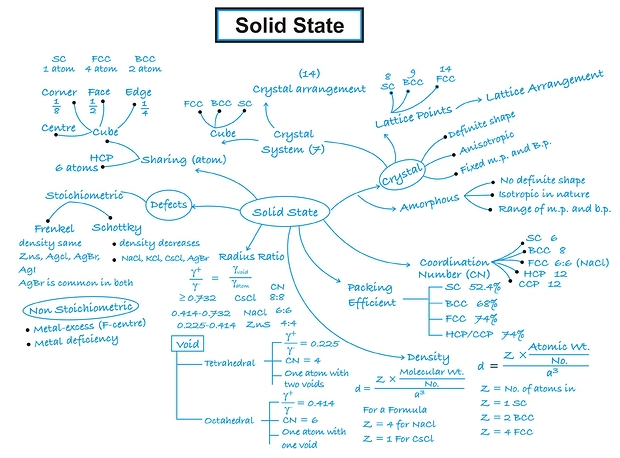Photo Electric Effect

Sir J.J. Thomson, observed that when a light of certain frequency strikes the surface of a metal, electrons are ejected from the metal. This phenomenon is known as photoelectric effect and the ejected electrons are called photoelectrons.

A few metals, which are having low ionisation energy like Cesium, show this effect under the action of visible light but many more show it under the action of more energetic ultraviolet light.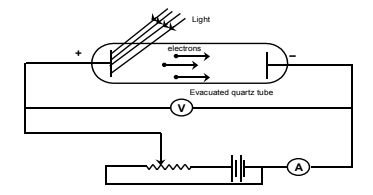An evacuated tube contains two electrodes connected to a source of variable voltage, with the metal plate whose surface is irradiated as the anode. Some of the photoelectrons that emerge from this surface have enough energy to reach the cathode despite its negative polarity, and they constitute the measured current. The slower photoelectrons are repelled before they get to the cathode. When the voltage is increased to a certain value Vo, of the order of several volts, no more photoelectrons arrive, as indicated by the current dropping to zero. This extinction voltage (or also referred as stopping potential) corresponds to the maximum photoelectron kinetic energy i.e., eVo = 1⁄2 mv2

The experimental findings are summarised as below:

i) Electrons come out as soon as the light (of sufficient energy) strikes the metal surface .

ii) The light of any frequency will not be able to cause ejection of electrons from a metal surface. There is a minimum frequency, called the threshold (or critical) frequency, which can just cause the ejection. This frequency varies with the nature of the metal. The higher the frequency of the light, the more energy the photoelectrons have. Blue light results in faster electrons than red light.

iii) Photoelectric current is increased with increase in intensity of light of same frequency, if emission is permitted i.e., a bright light yields more photoelectrons than a dim one of the same frequency, but the electron energies remain the same.

Light must have stream of energy particles or quanta of energy (hv). Suppose, the threshold frequency of light required to eject electrons from a metal is Vo, when a photon of light of this frequency strikes a metal it imparts its entire energy (hVo) to the electron.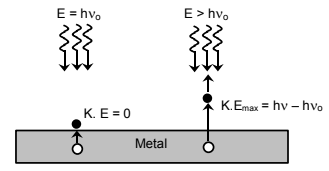“This energy enables the electron to break away from the atom by overcoming the attractive influence of the nucleus”. Thus each photon can eject one electron. If the frequency of light is less than Vo there is no ejection of electron. If the frequency of light is higher than Vo (let it be v), the photon of this light having higher energy (hv), will impart some energy to the electron that is needed to remove it away from the atom. The excess energy would give a certain velocity (i.e, kinetic energy) to the electron.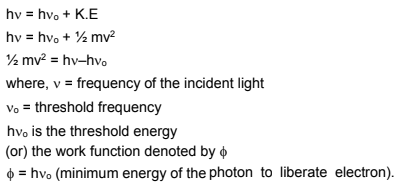It is constant for particular metal and is also equal to the ionization potential of gaseous atoms.

The kinetic energy of the photoelectrons increases linearly with the frequency of incident light. Thus, if the energy of the ejected electrons is plotted as a function of frequency, it result in a straight line whose slope is equal to Planck’s constant ‘h’ and whose intercept is hVo.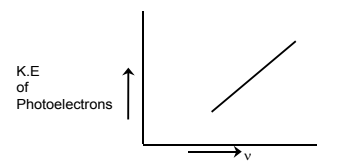Quantum Mechanical Model of atom

The atomic model which is based on the particle and wave nature of the electron is known as wave or quantum mechanical model of the atom. This was developed by Ervin Schrodinger in 1926. This model describes the electron as a three dimensioinal wave in the electronic field of positively charged nucleus. Schrodinger derived an equation which describes wave motion of an electron. The differential equation is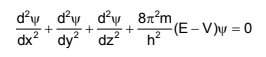where x, y, z are certain coordinates of the electron,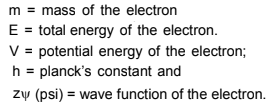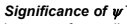The wave function may be regarded as the amplitude function expressed in terms of coordinates x, y and z. The wave function may have positive or negative values depending upon the value of coordinates. The main aim of Schrodinger equation is to give solution for probability approach. When the equation is solved, it is observed that for some regions of space the value of  is negative. But the probability must be always positive and cannot be negative, it is thus, proper to use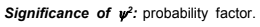It describes the probability of finding an electron within a small space. The space in which there is maximum probability of finding an electron is termed as orbital. The important point of the solution of the wave equation is that it provides a set of numbers called quantum numbers which describe energies of the electron in atoms, information about the shapes and orientations of the most probable distribution of electrons around nucleus.

Heisenberg’s Uncertainty Principle

All moving objects eg. car, ball, etc thrown in the air move along definite paths. Hence their position and velocity can be measured accurately at any instant of time.

As a cause of dual nature of matter, Heisenberg, gave a principle about the uncertainties in simultaneous measurement of position and momentum (mass x velocity) of small particles.

AS per this principle “It is impossible to measure simultaneously the position and momentum of a small microscopic moving particle with absolute accuracy or certainty” means, if an attempt is made to measure any one of these two quantities with higher accuracy, the other becomes less accurate.

The product of the uncertainty in position (x) and the uncertainty in the momentum (p) is equal to or greater than h/4 where h is the Planck’s constant.

p = m.v

where m is the mass of the particle and

v is the uncertainty in velocity

Thus, the mathematical expression for Heisenberg’s uncertainty principle is written as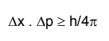Derivation of Angular Momentum from de Broglie Equation

According to Bohr’s model, the electron revolves around the nucleus in circular orbits.

According to de Broglie concept, the electron is not only a particle but has a wave character also.

If the wave is completely in phase, the circumference of the orbit must be equal to an integral multiple of wave length.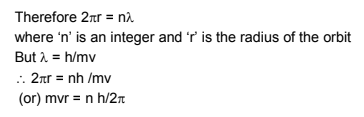which is Bohr’s postulate of angular momentum, where ‘n’ is the principal quantum number.

“Thus, the number of waves an electron makes in a particular Bohr orbit in one complete revolution is equal to the principal quantum number of the orbit”.

Alternatively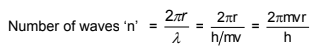where v and r are the velocity of electron and radius of that particular Bohr orbit in which number of waves are to be calculated, respectively.

The electron is revolving around the nucleus in a circular orbit. How many revolutions it can make in one second Let the velocity of electron be v m/sec. The distance it has to travel for one revolution 2r, (i.e., the circumference of the circle).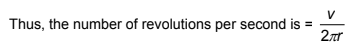Common unit of energy is electron volt which is amount of energy given when an electron is accelerated by a potential of exactly 1 volt. This energy equals the product of voltage and charge. Since in SI units coulombs  volts = Joules, 1 eV numerically equals the electronic charge except that joule replaces coulombs.

Dual Character - Particle and Wave Character of Matter and Radiation

In case of light some phenomenon like diffraction and interference can be explained on the basis of its wave character. However, the certain other phenomenon such as black body radiation and photoelectric effect can be explained only on the basis of its particle nature. Thus, light is said to have a dual character. Such studies on light were made by Einstein in 1905.

Louis de Broglie, in 1924 extended the idea of photons to material particles such as electron and he proposed that matter also has a dual character-as wave and as particle.

Derivation of de-Broglie equation

The wavelength of the wave associated with any material particle was calculated by analogy with photon.

In case of photon, if it is assumed to have wave character, its energy is given by